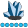## Length returned by PROC SQL's case statement should be as defined

Some functions truncate strings in PROC SQL:

``````data TEST;
length X \$300;
X=repeat('X',299);
run;

proc sql;
create table WANT as                            %* Var Len;
select trim(X)                     as TRIM      %* 300;
, translate(X,'a','b')        as TRANSLATE %* 300;
, scan(X,1)                   as SCAN      %* 300;
, left(X)                     as LEFT      %* 300;
, prxchange('s/a/b/',1,X)     as PRXCHANGE %* 200;
, cat(X)                      as CAT       %* 200;
, case when 1 then cat(X) end as CASE      %* 200;
from TEST;
quit;  ``````

But this can be fixed in some cases by forcing the variabel length:

``````proc sql;
create table WANT as                                          %* Str Len;
select trim(X)                       as TRIM       length=300 %* 300;
, translate(X,'a','b')          as TRANSLATE  length=300 %* 300;
, scan(X,1)                     as SCAN       length=300 %* 300;
, left(X)                       as LEFT       length=300 %* 300;
, prxchange('s/a/b/',1,X)       as PRXCHANGE  length=300 %* 300;
, cat(X)                        as CAT        length=300 %* 300;
, quote(X)                      as QUOTE      length=302 %* 302;
, case when 1 then quote(X) end as CASE       length=302 %*   0 <==;
from TEST;
quit;  ``````

The case statement however does not pass on the defined length to the executed function. It should.Lapis Lazuli | Level 10

I'm very interested to know more from a programming languages point of view (i.e., that of the software architect/developers). I can see why the length would be processed after the case logic. I'm not sure why you can't run

``, case when 1 then quote(X) length=302 end as CASE``

...moving the "length" statement into the case logic.Tourmaline | Level 20

Other issues that I wish were improved but do not belong to this discussion.
Just in case the head of PROC SQL development is looking. Wishful thinking...

1- Mentioning the length does not work for all functions. For example repeat() still trims at 200

2- Mentioning the length does not work with embedded functions. For example cat(cat(X)) fails to compute

3- Functions don't all behave the same way when option length= is not set. That's inconsistent.
trim() derives the length from its parameter while prxparse() trims to 200 while quote() returns a blank value.

4- In the second example, quote() works as expected but issues a NOTE: Invalid argument to function QUOTE.

5- More generally, 1) we need more coherence and 2) not being able to use functions on some strings in proc sql because values are trimmed is a painful limitation that should not be accepted.Tourmaline | Level 20

@paulkaefer

Thank you!

I reckon that the length should be carried to functions by proc sql rather than a language change, but any improvement is welcome!

This would allow proc sql to also carry the length not only when functions are embedded in a case clause, but also when functions are embedded in other functions.Tourmaline | Level 20

Also, to avoid repeating the length, the length= should be with the variable name, not with each case condition. This is particularly true if you have embedded cases.

Furthermore, length= is a non-ANSI SAS extension, so imho it is better located at the end, with the variable name, together with format= and label=, rather than scattered in a standard SQL case clauseSuper User

Looks to me like when they modified how PROC SQL handled strings longer than the old \$200 limit they missed some parts of the logic that handles CASE clauses.